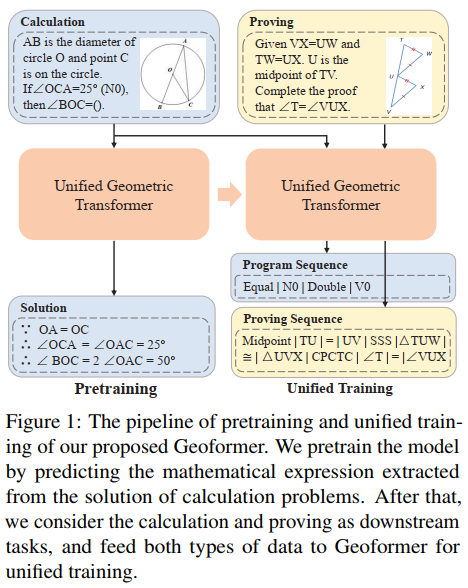EMNLP 2022
UniGeo: Unifying Geometry Logical Reasoning via Reformulating Mathematical Expression
Jiaqi Chen, Tong Li, Jinghui Qin, Pan Lu, Liang Lin, Chongyu Chen, Xiaodan Liang
EMNLP 2022

Abstract

Geometry problem solving is a well-recognized testbed for evaluating the high-level multimodal reasoning capability of deep models. In most existing works, two main geometry problems: calculation and proving, are usually treated as two specific tasks, hindering a deep model to unify its reasoning capability on multiple math tasks. However, in essence, these two tasks have similar problem representations and overlapped math knowledge which can improve the understanding and reasoning ability of a deep model on both two tasks. Therefore, we construct a large-scale Unified Geometry problem benchmark, UniGeo, which contains 4,998 calculation problems and 9,543 proving problems. Each proving problem is annotated with a multi-step proof with reasons and mathematical expressions. The proof can be easily reformulated as a proving sequence that shares the same formats with the annotated program sequence for calculation problems. Naturally, we also present a unified multitask Geometric Transformer framework, Geoformer, to tackle calculation and proving problems simultaneously in the form of sequence generation, which finally shows the reasoning ability can be improved on both two tasks by unifying formulation. Furthermore, we propose a Mathematical Expression Pretraining (MEP) method that aims to predict the mathematical expressions in the problem solution, thus improving the Geoformer model. Experiments on the UniGeo demonstrate that our proposed Geoformer obtains state-of-the-art performance by outperforming task-specific model NGS with over 5.6% and 3.2% accuracies on calculation and proving problems, respectively.

Framework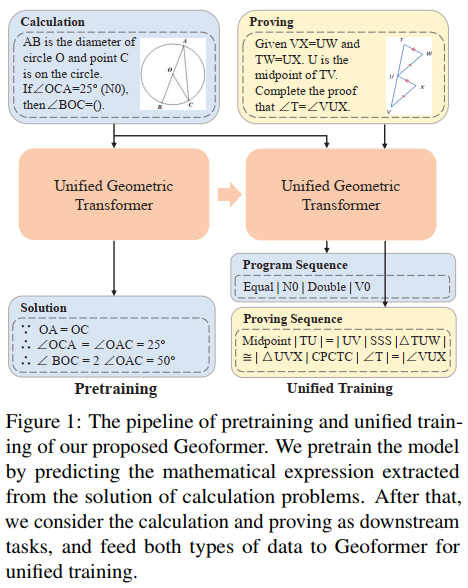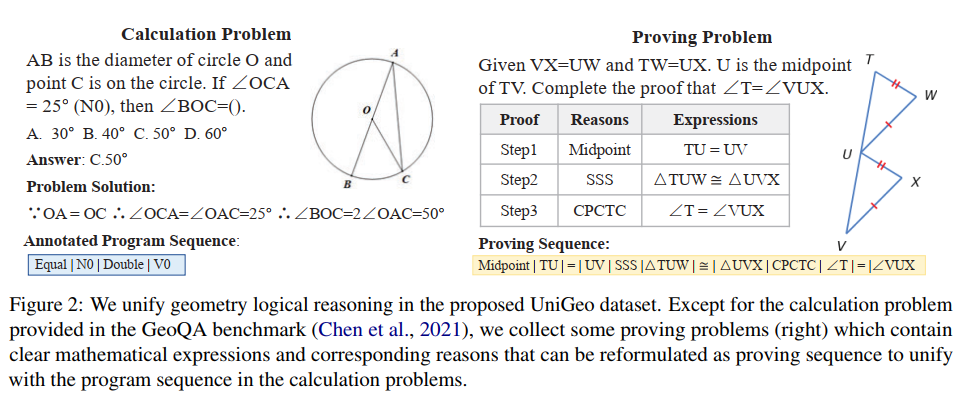Experiment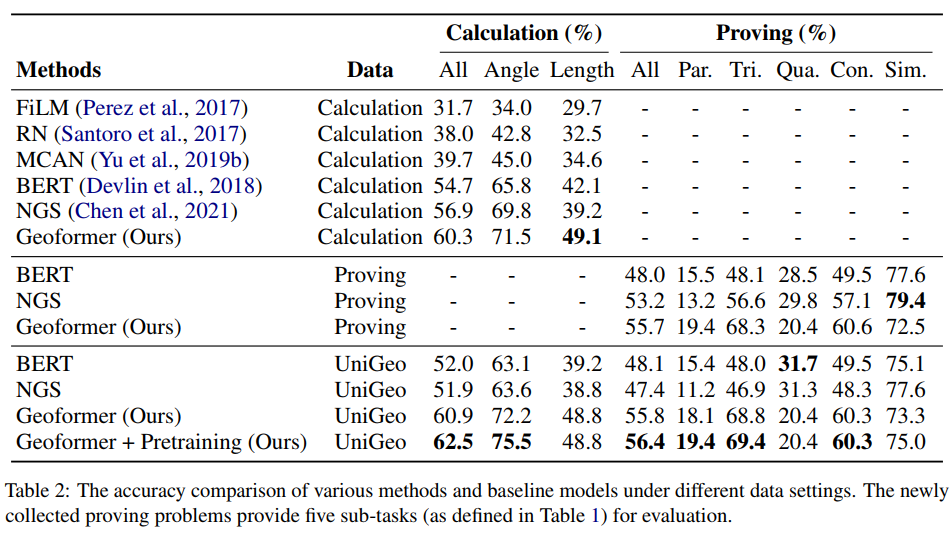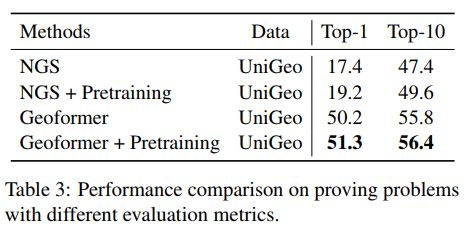Conclusion

Recently, geometry problem solving has attracted much attention in AI research while previous works mainly focus on geometry calculation problems. It is significant to explore the unified reasoning abilities of neural models on multiple math tasks. Therefore, we integrate geometry calculation and proving problems, and construct a unified geometry benchmark, UniGeo, containing 9,543 proving problems with proof reasons and mathematical expressions that can be reformulated as proving sequence to unify with the program sequence of calculation problems. We also propose a unified Geoformer that can address calculation and proving problems simultaneously. Besides, a mathematical expression pretraining way is proposed to promote the performance of the unified Geoformer. Experiments show that our Geoformer can well address two challenging geometry tasks with a single set of model weights, outperforming task-specialized models and obtaining state-of-the-art performance.